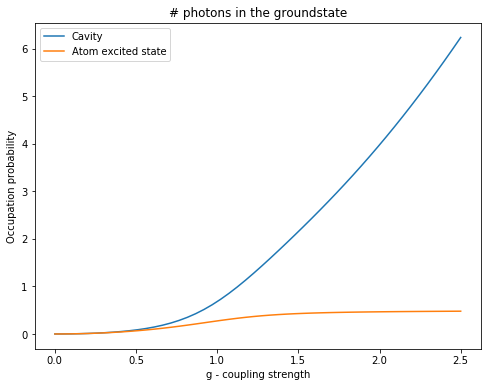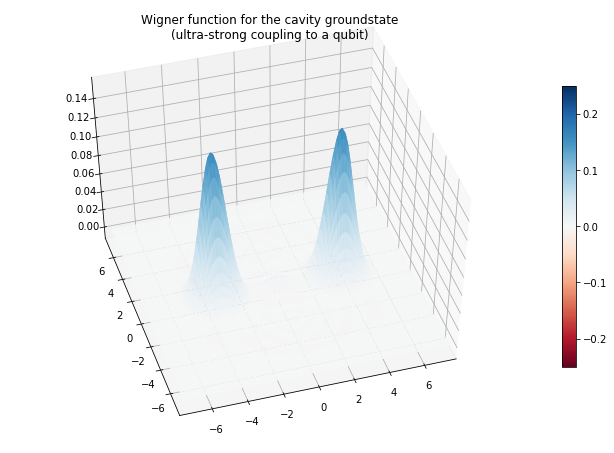QuTiP example: Groundstate of an ultra-strong coupled atom-cavity system¶

J.R. Johansson and P.D. Nation

In :
%matplotlib inline
In :
import matplotlib.pyplot as plt
In :
import numpy as np
In :
from qutip import *
import time
from mpl_toolkits.mplot3d import Axes3D
In :
def compute(N, wc, wa, glist, use_rwa):

# Pre-compute operators for the hamiltonian
a  = tensor(destroy(N), qeye(2))
sm = tensor(qeye(N), destroy(2))
nc = a.dag() * a
na = sm.dag() * sm

idx = 0
na_expt = np.zeros(shape(glist))
nc_expt = np.zeros(shape(glist))
for g in glist:

# recalculate the hamiltonian for each value of g
if use_rwa:
H = wc * nc + wa * na + g * (a.dag() * sm + a * sm.dag())
else:
H = wc * nc + wa * na + g * (a.dag() + a) * (sm + sm.dag())

# find the groundstate of the composite system
evals, ekets = H.eigenstates()
psi_gnd = ekets
na_expt[idx] = expect(na, psi_gnd)
nc_expt[idx] = expect(nc, psi_gnd)

idx += 1

return nc_expt, na_expt, ket2dm(psi_gnd)

#
# set up the calculation
#
wc = 1.0 * 2 * np.pi   # cavity frequency
wa = 1.0 * 2 * np.pi   # atom frequency
N = 20              # number of cavity fock states
use_rwa = False     # Set to True to see that non-RWA is necessary in this regime

glist = np.linspace(0, 2.5, 50) * 2 * np.pi # coupling strength vector

start_time = time.time()
nc, na, rhoss_final = compute(N, wc, wa, glist, use_rwa)
print('time elapsed = ' + str(time.time() - start_time))
time elapsed = 1.351557731628418
In :
#
# plot the cavity and atom occupation numbers as a function of
#
fig, ax = plt.subplots(figsize=(8,6))

ax.plot(glist/(2*np.pi), nc)
ax.plot(glist/(2*np.pi), na)
ax.legend(("Cavity", "Atom excited state"))
ax.set_xlabel('g - coupling strength')
ax.set_ylabel('Occupation probability')
ax.set_title('# photons in the groundstate');In :
#
# plot the cavity wigner function for the cavity state (final coupling strenght)
#
fig = plt.figure(2, figsize=(9, 6))
rho_cavity = ptrace(rhoss_final, 0)
xvec = np.linspace(-7.5,7.5,100)
X,Y = np.meshgrid(xvec, xvec)
W = wigner(rho_cavity, xvec, xvec)
ax = Axes3D(fig, azim=-107, elev=49)
surf=ax.plot_surface(X, Y, W, rstride=1, cstride=1, cmap=plt.cm.RdBu, alpha=1.0, linewidth=0.05,
vmax=0.25, vmin=-0.25)
ax.set_xlim3d(-7.5, 7.5)
ax.set_ylim3d(-7.5, 7.5)
fig.colorbar(surf, shrink=0.65, aspect=20)
ax.set_title("Wigner function for the cavity groundstate\n(ultra-strong coupling to a qubit)");Software version:¶

In :
from qutip.ipynbtools import version_table

version_table()
Out:
SoftwareVersion
QuTiP4.3.0.dev0+6e5b1d43
Numpy1.13.1
SciPy0.19.1
matplotlib2.0.2
Cython0.25.2
Number of CPUs2
BLAS InfoINTEL MKL
IPython6.1.0
Python3.6.2 |Anaconda custom (x86_64)| (default, Jul 20 2017, 13:14:59) [GCC 4.2.1 Compatible Apple LLVM 6.0 (clang-600.0.57)]
OSposix [darwin]
Thu Jul 20 23:01:57 2017 MDT